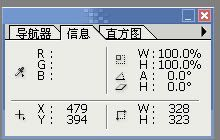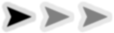krkr基础教程4

## 标签和按钮

1.判断一段对话有没有在游戏里被读过是判断的一个标签到另一个标签之间的部分
2.存档存的是标签的位置
3.游戏跳转是要跳到标签的位置

*标签名

*标签名|存档名

*test0
[position layer=message0 left=50 top=430 width=704 height=140 marginl=71 margint=17 marginr=73 marginb=17 opacity=0 visible=true frame="frame" page=fore]
[current layer=message0 pgae=fore]
[deffont face="黑体" size=20 color=0xffffff]
[defstyle linespacing=10 pitch=0 linesize=4][er]

[jump target=*test1]

*test1

*test0
[position layer=message0 left=50 top=430 width=704 height=140 marginl=71 margint=17 marginr=73 marginb=17 opacity=0 visible=true frame="frame" page=fore]
[current layer=message0 pgae=fore]
[deffont face="黑体" size=20 color=0xffffff][er]
[defstyle linespacing=10 pitch=0 linesize=4]

[call target=*test1]

[s]

*test1

[return]

*test0
[position layer=message0 left=50 top=430 width=704 height=140 marginl=71 margint=17 marginr=73 marginb=17 opacity=0 visible=true frame="frame" page=fore]
[current layer=message0 pgae=fore]
[deffont face="黑体" size=20 color=0xffffff]
[defstyle linespacing=10 pitch=0 linesize=4][er]

[s]

*test1
[er]

[s]

*test2
[er]

[s]

*test0
[position layer=message0 left=50 top=430 width=704 height=140 marginl=71 margint=17 marginr=73 marginb=17 opacity=0 visible=true frame="frame" page=fore]
[current layer=message0 pgae=fore]
[deffont face="黑体" size=20 color=0xffffff]
[defstyle linespacing=10 pitch=0 linesize=4][er]

[locate x=100 y=30]字会从locate指定的坐标开始出现[l][er]
[s]

*test1
[er]
50，20
[s]

*test2
[er]
70，50
[s]*test0
[position layer=message0 left=50 top=430 width=704 height=140 marginl=71 margint=17 marginr=73 marginb=17 opacity=0 visible=true frame="frame" page=fore]
[current layer=message0 pgae=fore]
[deffont face="黑体" size=20 color=0xffffff]
[defstyle linespacing=10 pitch=0 linesize=4][er]

[locate x=450 y=50]
[button graphic="button" target=*test1]
[s]

*test1
[er]

[s]

*test0
[position layer=message0 left=50 top=430 width=704 height=140 marginl=71 margint=17 marginr=73 marginb=17 opacity=0 visible=true frame="frame" page=fore]
[current layer=message0 pgae=fore]
[deffont face="黑体" size=20 color=0xffffff]
[defstyle linespacing=10 pitch=0 linesize=4][er]

[locate x=10 y=10]
[button graphic="button" target=*test1]
[s]

*test1
[locate x=10 y=30]
[button graphic="button" target=*test2]

[s]

*test2
[er]

*test0
[position layer=message0 left=50 top=430 width=704 height=140 marginl=71 margint=17 marginr=73 marginb=17 opacity=0 visible=true frame="frame" page=fore]
[current layer=message0 pgae=fore]
[deffont face="黑体" size=20 color=0xffffff]
[defstyle linespacing=10 pitch=0 linesize=4][er]

[current layer=message0 page=back]
[locate x=10 y=10]
[button graphic="button" target=*test1]
[s]

*test1
[current layer=message0 page=back][er]

*test0
[position layer=message0 left=50 top=430 width=704 height=140 marginl=71 margint=17 marginr=73 marginb=17 opacity=0 visible=true frame="frame" page=fore]
[current layer=message0 pgae=fore]
[deffont face="黑体" size=20 color=0xffffff]
[defstyle linespacing=10 pitch=0 linesize=4][er]

[locate x=50 y=110]
[button graphic="button" target=*test1]
[s]

*test1
[er]

[s]

jump参数详解
storage

target

countpage

call参数详解
storage

target

countpage

storage

target

exp

color

hint

onenter

onleave

countpage

clickse

clicksebuf

enterse

entersebuf

leavese

leavesebuf

button参数详解
graphic

normal

over

on

storage

target

recthit

exp

onenter

onleave

countpage

clickse

clicksebuf

enterse

entersebuf

leavese

leavesebuf

FrostMonarch

2016-07-20 13:51:07

aa